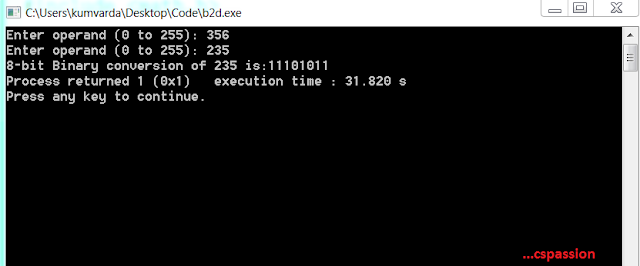## 8-Bit Decimal to Binary Conversion program in C

On 02:53 by Vardan Kumar in    No comments
Decimal to Binary Conversion

## Decimal to Binary Conversion

The most common number system we normally use in ff course decimal number system i.e. numbers from (0-9) but when it comes to computing class decimal number system is not really common rather computer systems use binary number system for computing purposes whether it is digital encoding or a discrete mathematics branch called Boolean Algebra. Hence once should be aware of the logic to convert a decimal number system to its respective binary equivalent.

### Logic

We will use the theoretical approach as its programmatic implementation to fulfill our desired purpose of converting decimal number system to its binary twin. Also we'll fetch our result bit by bit so we will use an array to store the bits.

1. So first of all we'll take our operand and divide it with two.
2. We'll store the remainder as our result bit in the array.
3. And we will loop through till our operand is greater than 0.
This was the computation logic. Note in order to display the result we'll traverse the array in reverse order and print the result bit by bit. Also we can modify the number of bits to our desired number, We recommend use of macros for that purpose.Output:Decimal to Binary Conversion

### Source Code

#include <stdio.h>
#include <math.h>

void Decimal2Binary(int op, int bop[]){
int result, i = 0;
do{
result = op % 2;
op /= 2;
bop[i] = result;
i++;
}while(op > 0);
}
void main()
{

int op,i;
int  aOp = {0,0,0,0,0,0,0,0};

printf("Enter operand (0 to 255): ");
scanf("%d", &op);
while(op < 0 || op > 255)
{
printf("Enter operand (0 to 255): ");
scanf("%d", &op);
}
Decimal2Binary(op, aOp);
printf("8-bit Binary conversion of %d is:",op);
for(i=7;i>=0;i--)
{
printf("%d",aOp[i]);
}
}

Also read 8-Bit Binary to Decimal Conversion